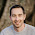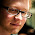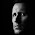# Go deh!

Mainly Tech projects on Python and Electronic Design Automation.

## Monday, March 25, 2013

### itertools.first ?

Note: Extended with Stop Press! section.

Note: Extended later with "Identity with next" section.
Note: Extended later with "Deviant" section.

I wrote something on Rosetta Code and found myself wanting, (again), a version of the any() function that returned the first True elements value rather than the Boolean value True.

The code was calculating the Harshad numbers defined as a positive integer that is divisible by the sum of its digits. I need to show the first 20 and the one greater than 1000.

I came up with the following:

```>>> import itertools
n=1
while True:
if n % sum(int(ch) for ch in str(n)) == 0:
yield n
n += 1

>>> list(itertools.islice(harshad(), 0, 22))
[1, 2, 3, 4, 5, 6, 7, 8, 9, 10, 12, 18, 20, 21, 24, 27, 30, 36, 40, 42, 45, 48]
>>> for n in harshad():
if n > 1000:
print(n)
break

1002
```

It does the job, but I wanted a more functional way of finding the 1000'th element.

I tried to use any but was stuck because it only returns True or False. I could make it work :

```>>> tmp = []
>>> any((tmp.append(x), ) for x in harshad() if x > 1000)
True
>>> tmp
1002
>>>```

But the above is an obscuring hack to get the value of x out of the genexp.

### Needed

What I need is a function that I will call first, that works like any but returns the first value that is true (rather than True) on success, otherwise False.

If any can be defined similarly to:

```def any(iterable):
for value in iterable:
if value:
return True
return False
```

Then function first would be defined as:

```def first(iterable):
for value in iterable:
if value:
return value
return False```

And I could then have written:

```>>> first(x for x in harshad() if x > 1000)
1002
>>>```

Which is so clean I would think its a candidate for adding to the itertools module.

What do you think?

(I'll add this to Reddit and g+ for discussion as well).

## Stop Press!

Darn! I have just worked out that I could have written the following:

```>>> (x for x in harshad() if x > 1000).__next__()
1002
>>>```

It works and is not so bad looking. Not as good as a first() function, but usable.

### Identity with next

aceofears on Reddit pointed me in the direction of the default value for the next() function. That got me thinking and I think I have an identity between my first() and the existing next()

first(iterator)  next((item for item in iterator if item),
False)

If the above holds then I know I'd rather write first(), but could live without it {melodramatic sigh}.

Oh, these should hold too:

any(iterator)  next((True for item in iterator if item),
False)

all(iterator)  next((False for item in iterator if not item),
True)

### Deviant

There is an identy missing. any() is to first() as all() is to ???
I would call this missing function deviant(). Its action would be to return the first item from an iterator that was false rather than the Boolean False.

So:

deviant(iterator)  next((item for item in iterator if not item),
True)

(I have not worked out a use case for this as yet though).

1.Why not just:

next(x for x in harshad() if x > 1000)

Edit: Missed your edit, this doesn't add anything sorry!

1.Yep. I've used next before. But I was blinded by the way I was using it and just could not see that this was another use case! Well I've been up for over 18 hours and should not have blogged when tired. This will teach me.

Thanks.

2.Why dont you just use next? This should work:
>>> next(x for x in harshad() if x > 1000)

it even works if your iterable returns False:
>>> xs = (None, False, True)
>>> next(x for x in xs if x is not None)

3.FTR, there is still a valid need for a first and there is also a solution: https://crate.io/packages/first/

1.Hey hynek. Thanks for the link.
I liked the regecp example but I am not convinced that the default and key arguments are necessary and would prefer first is kept short & sweet. Instead of that:

first([1, 1, 3, 4, 5], key=lambda x: x % 2 == 0)

I would prefer the equivalent:

first(x for x in [1, 1, 3, 4, 5] if not x % 2)

4.You don't need first value _that_ is true, you need first value for which _predicate_ is true. That is just next(filter(predicate, iterator)). And if by chance you really need the value being true, that's next(filter(None, iterator)).

for deviant, you just need filterfalse (from itertools). But as you noticed, it's a lot less useful, since there are much fewer interesting objects that are false than true.

1.My definition of first would be much easier to understand and simpler to write for the use case given, although yours could work too.